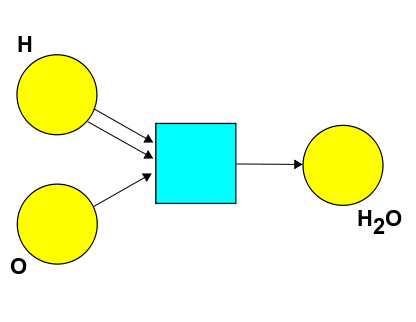Robert wrote:

> I'm still not sure I know what John meant, I'll have to try again later.

In Puzzle 68 I was asking this. Suppose there's just one chemical reaction you can do:When can you turn

$a \text{H} + b \text{O} + c \text{H}_2\text{O}$

into

$a' \text{H} + b' \text{O} + c' \text{H}_2\text{O}$

using this reaction? Here \$$a,b,c\$$ and \$$a',b',c'\$$ are natural numbers.

For example, you _can_ turn

$4 \text{H} + 2 \text{O} + \text{H}_2\text{O}$

into

$3 \text{H}_2\text{O}$

using this reaction. (Of course you have to use it twice, but that's okay.) You _cannot_ turn

$3 \text{H} + 2 \text{O} + \text{H}_2\text{O}$

into

$\text{O} + 2 \text{H}_2\text{O}$

using this reaction. So, what's the general rule? If you want, you can abbreviate

$a \text{H} + b \text{O} + c \text{H}_2\text{O}$

as a list of 3 natural numbers \$$(a,b,c) \$$, and write

$(a',b',c') \le (a,b,c)$

to mean "you can turn \$$a \text{H} + b \text{O} + c \text{H}_2\text{O} \$$ into
\$$a' \text{H} + b' \text{O} + c' \text{H}_2\text{O} \$$."

Michael Hong tried answering this question in Comment 4:

> **Puzzle 68.** Define \$$(a, b, c) \leq (a', b', c')\$$ if and only if \$$a \gt a', b \gt b' \, and \, c \lt c'\$$.

This is the right _kind_ of answer, but it's not the right answer. If this were true, we would have \$$(1,1,0) \le (0,0,1) \$$, meaning we could turn \$$\text{H} + \text{O}\$$ into \$$\text{H}_2\text{O}\$$. That would be cool, but actually we can't do that using this reaction:Marius gives an interesting alternative answer in Comment 8. Do you think this answer is correct?